set.seed(12345) # for reproducibility
options(knitr.kable.NA = '')

# Some packages need to be loaded. We use pacman as a package manager, which takes care of the other packages.
if (!require("pacman", quietly = TRUE)) install.packages("pacman")
if (!require("Rmisc", quietly = TRUE)) install.packages("Rmisc") # Never load it directly.
pacman::p_load(tidyverse, knitr, car, afex, emmeans, parallel, ordinal,
ggbeeswarm, RVAideMemoire)

klippy::klippy()               

# 1 Day 1 Familiarization

Forty eight non-famous faces were repeated 30 times on Day 1. Each participant (13 in total) completed 10 blocks of 144 trials each (1,440 trials in total). Each face was repeated three times within a block. In each trial, participants indicated whether it was the first, second, or third time the given face was presented within the block.

D1 <- read.csv("data/data_FamSM_Exp3A_Face_PRE.csv", header = T)
D1$SID <- factor(D1$SID)
glimpse(D1, width=70)
## Observations: 18,720
## Variables: 8
## $SID <fct> 1, 1, 1, 1, 1, 1, 1, 1, 1, 1, 1, 1, 1, 1, 1, 1, 1, … ##$ Block   <int> 1, 1, 1, 1, 1, 1, 1, 1, 1, 1, 1, 1, 1, 1, 1, 1, 1, …
## $Trial <int> 1, 2, 3, 4, 5, 6, 7, 8, 9, 10, 11, 12, 13, 14, 15, … ##$ RepTime <int> 1, 1, 1, 1, 1, 1, 1, 1, 2, 1, 1, 1, 1, 1, 1, 3, 1, …
## $Resp <int> 0, 0, 0, 0, 0, 0, 0, 0, 0, 0, 0, 0, 0, 0, 0, 1, 0, … ##$ Corr    <int> 0, 0, 0, 0, 0, 0, 0, 0, 0, 0, 0, 0, 0, 0, 0, 0, 0, …
## $RT <dbl> 0.00, 0.00, 0.00, 0.00, 0.00, 0.00, 0.00, 0.00, 0.0… ##$ ImgName <fct> unfamfemale17.jpg, capture002 copy.jpg, capture017 …
# 1. SID: participant ID
# 2. Block: 1~10
# 3. Trial: 1~144
# 4. RepTime: 3 repetitions per image per block. 1~3
# 5. Resp: repetition counting, 1~3, 0 = no response
# 6. Corr: correctness, 1 = correct, 0 = incorrect or no response
# 7. RT: reaction times in ms.
# 8. ImgName: name of stimuli

table(D1$SID) ## ## 1 2 3 4 5 6 7 8 9 10 11 12 13 ## 1440 1440 1440 1440 1440 1440 1440 1440 1440 1440 1440 1440 1440 ## 1.1 Accuracy & RT # all blocks cD1s <- D1 %>% group_by(SID) %>% summarise(M = mean(Corr)*100) %>% ungroup() # each block cD1slong <- D1 %>% group_by(SID, Block) %>% summarise(Accuracy = mean(Corr)*100) %>% ungroup() cD1g <- Rmisc::summarySEwithin(data=cD1slong, measurevar = "Accuracy", idvar = "SID", withinvars = "Block") h1 <- ggplot(cD1g, mapping=aes(x=Block, y=Accuracy, group=1)) + geom_ribbon(aes(ymin=Accuracy-ci, ymax=Accuracy+ci), fill="darkred", alpha=0.3) + geom_line(colour="darkred", size = 1) + geom_line(cD1slong, alpha = 0.3, show.legend = FALSE, mapping=aes(x=Block, y=Accuracy, group=SID, color=SID)) + coord_cartesian(ylim = c(0, 100), clip = "on") + labs(x = "Block", y = "Accuracy (%)") + scale_x_discrete(breaks=seq(1,10,1)) + theme_bw(base_size = 18) + theme(panel.grid.major = element_blank(), panel.grid.minor = element_blank(), legend.position = "none") rD1slong <- D1 %>% filter(Corr==1) %>% group_by(SID, Block) %>% summarise(RT = mean(RT)) %>% ungroup() rD1g <- Rmisc::summarySEwithin(data=rD1slong, measurevar = "RT", idvar = "SID", withinvars = "Block") h2 <- ggplot(rD1g, mapping=aes(x=Block, y=RT, group=1)) + geom_ribbon(aes(ymin=RT-ci, ymax=RT+ci), fill="darkred", alpha=0.3) + geom_line(colour="darkred", size = 1) + geom_line(rD1slong, alpha = 0.3, show.legend = FALSE, mapping=aes(x=Block, y=RT, group=SID, color=SID)) + coord_cartesian(ylim = c(0, 800), clip = "on") + labs(x = "Block", y = "Correct RT (ms)") + scale_x_discrete(breaks=seq(1,10,1)) + theme_bw(base_size = 18) + theme(panel.grid.major = element_blank(), panel.grid.minor = element_blank(), legend.position = "none") h1 + h2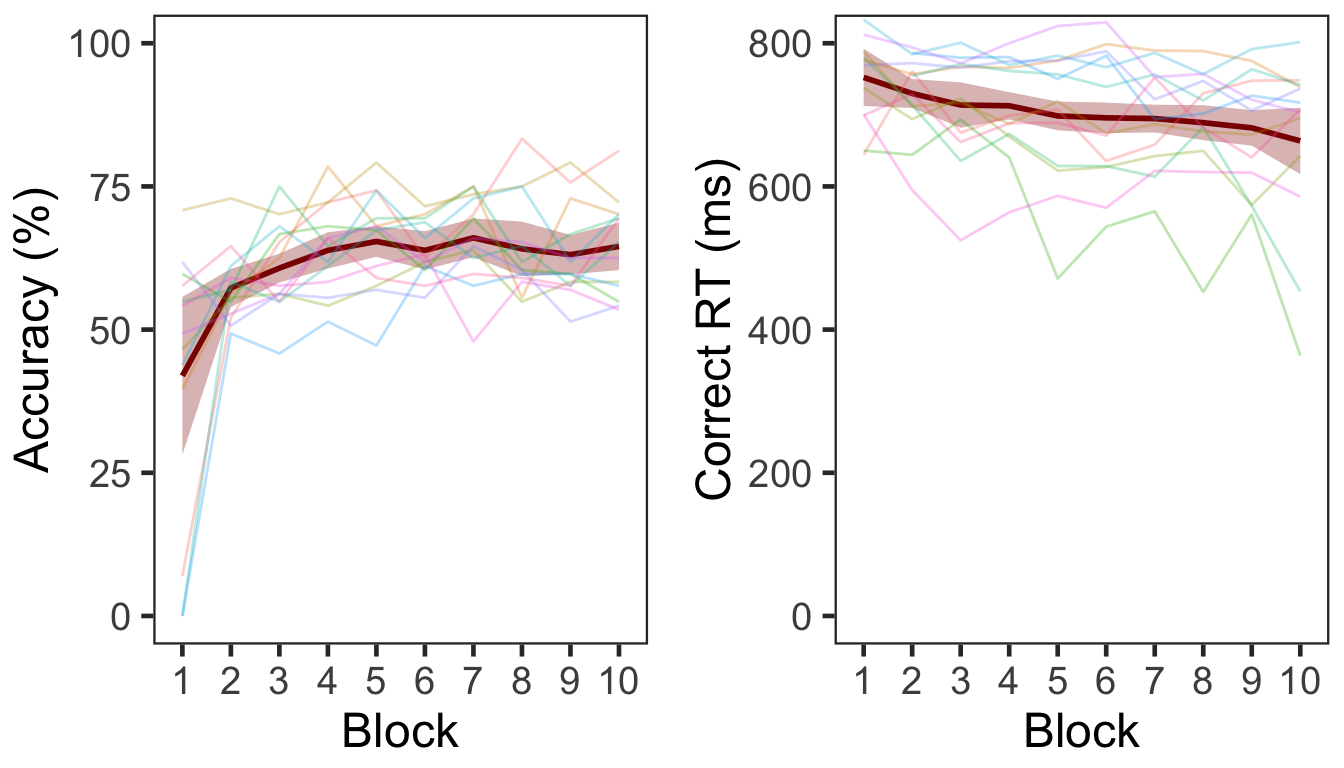Three participants (1st, 7th, & 9th) did not make responses in all or most of trials in Block 1. The performance improved over time; accuracy increased and RT decreased in subsequent blocks. Overall accuracy was 61.07% (s.d. = 6.18). # 2 Item Repetition Phase The same three-phase procedure as in Experiments 1 and 2 was conducted on Day 2. In the first phase, 24 out of the 48 non-famous faces learned on Day 1 were repeated eight times, resulting in 192 trials in total. In each trial, participants made a male/female judgment. P1 <- read.csv("data/data_FamSM_Exp3A_Face_REP.csv", header = T) glimpse(P1, width=70) ## Observations: 2,496 ## Variables: 8 ##$ SID     <int> 1, 1, 1, 1, 1, 1, 1, 1, 1, 1, 1, 1, 1, 1, 1, 1, 1, …
## $RepTime <int> 1, 1, 1, 1, 1, 1, 1, 1, 1, 1, 1, 1, 1, 1, 1, 1, 1, … ##$ Trial   <int> 1, 2, 3, 4, 5, 6, 7, 8, 9, 10, 11, 12, 13, 14, 15, …
## $ImgCat <int> 2, 1, 1, 2, 2, 1, 1, 2, 1, 2, 1, 1, 1, 2, 1, 2, 2, … ##$ Resp    <int> 1, 1, 1, 1, 2, 1, 1, 2, 1, 2, 1, 1, 1, 2, 1, 2, 2, …
## $Corr <int> 0, 1, 1, 0, 1, 1, 1, 1, 1, 1, 1, 1, 1, 1, 1, 1, 1, … ##$ RT      <dbl> 699.85, 511.25, 440.06, 392.80, 489.64, 506.39, 531…
## $ImgName <fct> unfamfemale37.jpg, capture010 copy.jpg, unfammale08… # 1. SID: participant ID # 2. RepTime: number of repetition, 1~8 # 3. Trial: 1~24 # 4. ImgCat: stimulus category. male vs. female # 5. Resp: male/female judgment, 1 = male, 2 = female, 0 = no response # 6. Corr: correctness, 1 = correct, 0 = incorrect or no response # 7. RT: reaction times in ms. # 8. ImgName: name of stimuli table(P1$SID)
##
##   1   2   3   4   5   6   7   8   9  10  11  12  13
## 192 192 192 192 192 192 192 192 192 192 192 192 192

## 2.1 Accuracy

The mean accuracy of the male/female judgment task was 97.28%. In the figure below, the red point and error bars represent the mean and 95% bootstrapped CIs.

P1slong <- P1 %>% group_by(SID) %>%
summarise(Accuracy = mean(Corr)*100) %>%
ungroup()

ggplot(data=P1slong, aes(x=1, y=Accuracy)) +
geom_violin(width = 1, trim = TRUE) +
ggbeeswarm::geom_quasirandom(dodge.width = 0.7, color = "blue", size = 3, alpha = 0.2,
show.legend = FALSE) +
stat_summary(fun.data = "mean_cl_boot", color = "darkred", size = 1) +
coord_flip(ylim = c(50, 100), clip = "on") +
labs(y = "Male/Female Judgment Accuracy (%)") +
theme_bw(base_size = 18) +
theme(panel.grid.major = element_blank(),
panel.grid.minor = element_blank(),
axis.title.y = element_blank(),
axis.ticks.y = element_blank(),
axis.text.y = element_blank(),
aspect.ratio = .3)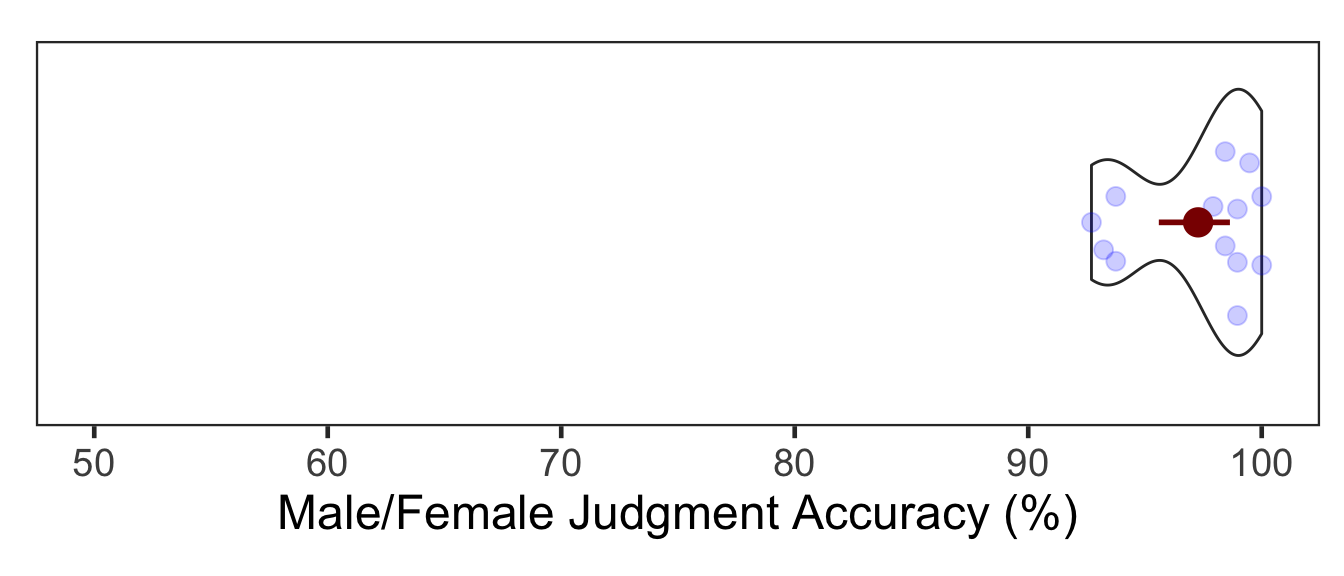# 3 Item-Source Association Phase

Participants learned 48 face-location (a quadrant on the screen) associations. They were instructed to pay attention to the location of each face while making a male/female judgment. All faces shown on Day 1 were presented once in one of four quadrants. Item repetition was the single within-participant independent variable. Half of the faces had been repeated in the first phase (repeated) while the other half had not (unrepeated).

P2 <- read.csv("data/data_FamSM_Exp3A_Face_SRC.csv", header = T)
P2$Repetition = factor(P2$Repetition, levels=c(1,2), labels=c("Repeated","Unrepeated"))

glimpse(P2, width=70)
## Observations: 624
## Variables: 9
## $SID <int> 1, 1, 1, 1, 1, 1, 1, 1, 1, 1, 1, 1, 1, 1, 1, 1, … ##$ Trial      <int> 1, 2, 3, 4, 5, 6, 7, 8, 9, 10, 11, 12, 13, 14, 1…
## $Repetition <fct> Repeated, Repeated, Repeated, Unrepeated, Unrepe… ##$ ImgCat     <int> 1, 2, 2, 1, 1, 1, 1, 2, 2, 1, 2, 2, 2, 2, 1, 2, …
## $Loc <int> 1, 2, 1, 3, 4, 1, 2, 4, 1, 4, 2, 3, 3, 2, 3, 3, … ##$ Resp       <int> 1, 2, 1, 1, 1, 1, 1, 2, 2, 1, 2, 2, 2, 2, 1, 2, …
## $Corr <int> 1, 1, 0, 1, 1, 1, 1, 1, 1, 1, 1, 1, 1, 1, 1, 1, … ##$ RT         <dbl> 746.56, 726.74, 875.32, 831.62, 740.08, 752.38, …
## $ImgName <fct> unfammale05.jpg, capture015 copy.jpg, unfamfemal… # 1. SID: participant ID # 2. Trial: 1~48 # 3. Repetition: 1 = repetition, 2 = unrepeated # 4. ImgCat: stimulus category. male vs. female # 5. Loc: location (source) of memory item; quadrants, 1~4 # 6. Resp: male/female judgment, 1 = male, 2 = female, 0 = no response # 7. Corr: correctness, 1 = correct, 0 = incorrect or no response # 8. RT: reaction times in ms. # 9. ImgName: name of stimuli table(P2$Repetition, P2$SID) ## ## 1 2 3 4 5 6 7 8 9 10 11 12 13 ## Repeated 24 24 24 24 24 24 24 24 24 24 24 24 24 ## Unrepeated 24 24 24 24 24 24 24 24 24 24 24 24 24 ## 3.1 Accuracy We calculated the mean and s.d. of individual participants’ accuracy for each condition. There was only negligible difference between the repeated vs. unrepeated conditions. In the following plot, red points and error bars represent the means and 95% CIs. # phase 1, subject-level, long-format P2slong <- P2 %>% group_by(SID, Repetition) %>% summarise(Accuracy = mean(Corr)*100) %>% ungroup() # summary table P2g <- P2slong %>% group_by(Repetition) %>% summarise(M = mean(Accuracy), SD = sd(Accuracy)) %>% ungroup() P2g %>% kable() Repetition M SD Repeated 96.15385 4.948092 Unrepeated 96.47436 6.981738 # group level, needed for printing & geom_pointrange # Rmisc must be called indirectly due to incompatibility between plyr and dplyr. P2g$ci <- Rmisc::summarySEwithin(data = P2slong, measurevar = "Accuracy", idvar = "SID", withinvars = "Repetition")$ci P2g$Accuracy <- P2g$M ggplot(P2slong, aes(x=Repetition, y=Accuracy, fill=Repetition)) + geom_violin(width = 0.5, trim=TRUE) + ggbeeswarm::geom_quasirandom(color = "blue", size = 3, alpha = 0.2, width = 0.2, show.legend = FALSE) + geom_pointrange(P2g, inherit.aes=FALSE, mapping=aes(x = Repetition, y=Accuracy, ymin = Accuracy - ci, ymax = Accuracy + ci), colour="darkred", size = 1) + coord_cartesian(ylim = c(50, 100), clip = "on") + labs(x = "Item Repetition", y = "Male/Female Judgment \n Accuracy (%)") + scale_fill_manual(values=c("#E69F00", "#56B4E9"), labels=c("Repeated", "Unrepeated")) + theme_bw(base_size = 18) + theme(panel.grid.major = element_blank(), panel.grid.minor = element_blank(), legend.position = "none")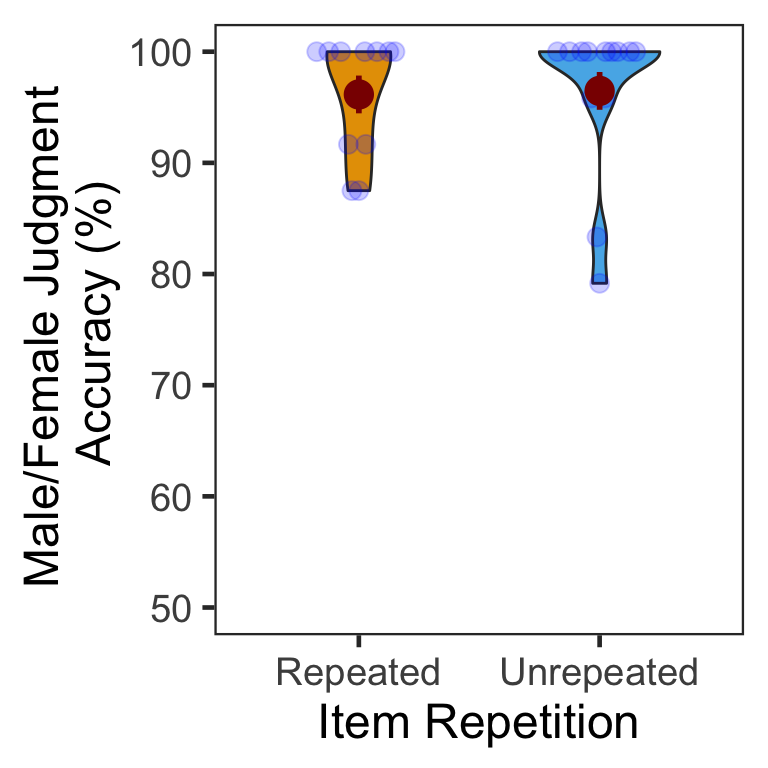### 3.1.1 ANOVA Mean percentage accuracy was submitted to a one-way repeated measures ANOVA. The effect of item repetition was not statistically significant. p2.aov <- aov_ez(id = "SID", dv = "Accuracy", data = P2slong, within = "Repetition") anova(p2.aov, es = "pes") %>% kable(digits = 4) num Df den Df MSE F pes Pr(>F) Repetition 1 12 7.9015 0.0845 0.007 0.7762 # 4 Source Memory Test Phase In each trial, participants first indicated in which quadrant a given face appeared during the item-source association phase. Participants then rated how confident they were about their memory judgment. P3 <- read.csv("data/data_FamSM_Exp3A_Face_TST.csv", header = T) P3$Repetition = factor(P3$Repetition, levels=c(1,2), labels=c("Repeated","Unrepeated")) glimpse(P3, width=70) ## Observations: 624 ## Variables: 9 ##$ SID        <int> 1, 1, 1, 1, 1, 1, 1, 1, 1, 1, 1, 1, 1, 1, 1, 1, …
## $Trial <int> 1, 2, 3, 4, 5, 6, 7, 8, 9, 10, 11, 12, 13, 14, 1… ##$ Repetition <fct> Unrepeated, Unrepeated, Unrepeated, Repeated, Re…
## $AscLoc <int> 2, 3, 2, 4, 2, 4, 4, 4, 4, 4, 1, 1, 3, 3, 3, 2, … ##$ ScrResp    <int> 2, 3, 3, 4, 2, 4, 4, 4, 4, 4, 1, 1, 3, 3, 2, 2, …
## $Corr <int> 1, 1, 0, 1, 1, 1, 1, 1, 1, 1, 1, 1, 1, 1, 0, 1, … ##$ RT         <dbl> 6550.50, 1672.18, 6290.12, 2153.03, 5672.27, 166…
## $Confident <int> 1, 4, 2, 4, 1, 2, 4, 4, 4, 4, 4, 4, 3, 4, 2, 4, … ##$ ImgName    <fct> unfammale09.jpg, unfamfemale06.jpg, capture011 c…
# 1. SID: participant ID
# 2. Trial: 1~48
# 3. Repetition: 1 = repetition, 2 = unrepeated
# 4. AscLoc: location (source) in which the item was presented in Phase 2; quadrants, 1~4
# 5. SrcResp: source response; quadrants, 1~4
# 6. Corr: correctness, 1 = correct, 0 = incorrect
# 7. RT: reaction times in ms.
# 8. Confident: confidence rating, 1~4
# 9. ImgName: name of stimuli

table(P3$Repetition, P3$SID)
##
##               1  2  3  4  5  6  7  8  9 10 11 12 13
##   Repeated   24 24 24 24 24 24 24 24 24 24 24 24 24
##   Unrepeated 24 24 24 24 24 24 24 24 24 24 24 24 24

## 4.1 Accuracy

We calculated the mean and s.d. of individual participants’ mean percentage accuracy. In the following plot, red points and error bars represent the means and 95% within-participants CIs.

# phase 3, subject-level, long-format
P3ACCslong <- P3 %>% group_by(SID, Repetition) %>%
summarise(Accuracy = mean(Corr)*100) %>%
ungroup()

# summary table
P3ACCg <- P3ACCslong %>% group_by(Repetition) %>%
summarise(M = mean(Accuracy), SD = sd(Accuracy)) %>%
ungroup()
P3ACCg %>% kable()
Repetition M SD
Repeated 54.16667 13.50154
Unrepeated 65.06410 18.82996
# wide format, needed for geom_segments.
P3ACCswide <- P3ACCslong %>% spread(key = Repetition, value = Accuracy)

# group level, needed for printing & geom_pointrange
# Rmisc must be called indirectly due to incompatibility between plyr and dplyr.
P3ACCg$ci <- Rmisc::summarySEwithin(data = P3ACCslong, measurevar = "Accuracy", idvar = "SID", withinvars = "Repetition")$ci
P3ACCg$Accuracy <- P3ACCg$M

ggplot(data=P3ACCslong, aes(x=Repetition, y=Accuracy, fill=Repetition)) +
geom_violin(width = 0.5, trim=TRUE) +
geom_point(position=position_dodge(0.5), color="gray80", size=1.8, show.legend = FALSE) +
geom_segment(data=P3ACCswide, inherit.aes = FALSE,
aes(x=1, y=P3ACCswide$Repeated, xend=2, yend=P3ACCswide$Unrepeated), color="gray80") +
geom_pointrange(data=P3ACCg,
aes(x = Repetition, ymin = Accuracy-ci, ymax = Accuracy+ci, group = Repetition),
position = position_dodge(0.5), color = "darkred", size = 1, show.legend = FALSE) +
scale_fill_manual(values=c("#E69F00", "#56B4E9"),
labels=c("Repeated", "Unrepeated")) +
labs(x = "Item Repetition",
y = "Source Memory Accuracy (%)") +
coord_cartesian(ylim = c(0, 100), clip = "on") +
theme_bw(base_size = 18) +
theme(panel.grid.major = element_blank(),
panel.grid.minor = element_blank(),
legend.position = "none")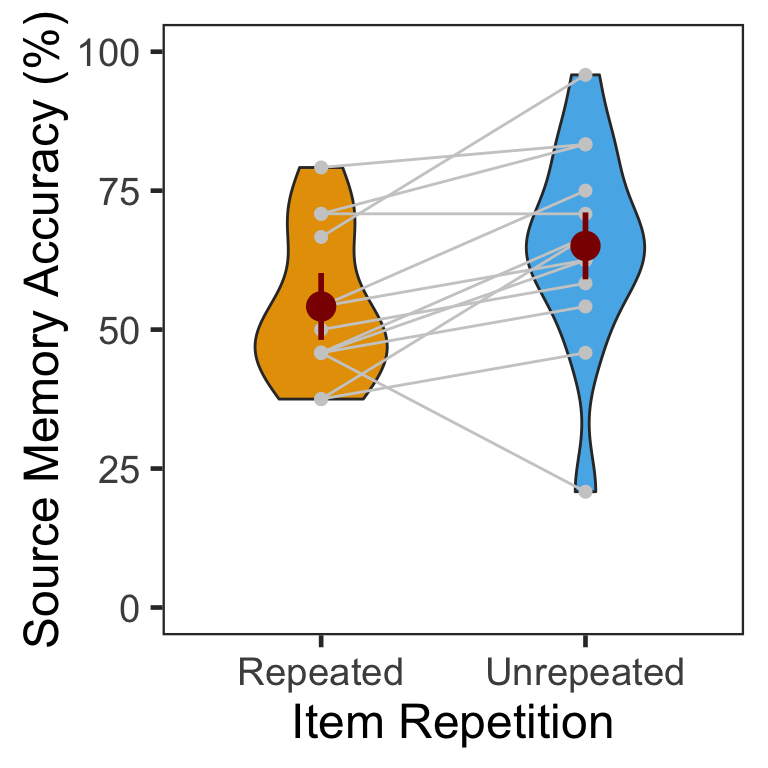Source memory accuracy was greater in the unrepeated than repeated condition (novelty benefit).

### 4.1.1 ANOVA

Mean percentage accuracy was submitted to a one-way repeated measures ANOVA.

ci95 <- P3ACCswide %>%
mutate(Diff = Unrepeated - Repeated) %>%
summarise(lower = mean(Diff) - qt(0.975,df=n()-1)*sd(Diff)/sqrt(n()),
upper = mean(Diff) + qt(0.975,df=n()-1)*sd(Diff)/sqrt(n()))

p3.corr.aov <- aov_ez(id = "SID", dv = "Accuracy", data = P3ACCslong, within = "Repetition")
anova(p3.corr.aov, es = "pes") %>% kable(digits = 4)
num Df den Df MSE F pes Pr(>F)
Repetition 1 12 99.1587 7.7845 0.3935 0.0163

Source memory accuracy was higher for unrepeated than repeated faces. The 95% CI of difference between the means was [2.39, 19.41].

### 4.1.2 GLMM

To supplement conventional ANOVAs, we tested generalized linear mixed models (GLMM) on source memory accuracy. This mixed modeling approach with a binomial link function is expected to properly handle binary data such as source memory responses (i.e., correct or not; Jaeger, 2008).

The full model (full1) was built with a fixed effect (item repetition). The model was maximal in that it included both by-participant and by-item random intercepts, and by-participant random slopes for item repetition (Barr, Levy, Scheepers, & Tily, 2013). In case the maximal model does not converge successfully, we built another model (full2) with the maximal random structure but with the correlations among the random terms removed (Singmann, 2018).

To fit the models, we used the mixed() of the afex package (Singmann, Bolker, & Westfall, 2017) which was built on the lmer() of the lme4 package (Bates, Maechler, Bolker, & Walker, 2015). The mixed() assessed the statistical significance of fixed effects by comparing a model with the effect in question against its nested model which lacked the effect in question. P-values of the effects were obtained by likelihood ratio tests (LRT).

(nc <- detectCores())
cl <- makeCluster(rep("localhost", nc))

full1 <- mixed(Corr ~ Repetition + (Repetition|SID) + (1|ImgName),
P3, method = "LRT", cl = cl,
control = glmerControl(optCtrl = list(maxfun = 1e6)))
full2 <- mixed(Corr ~ Repetition + (Repetition||SID) + (1|ImgName),
P3, method = "LRT", cl = cl,
control = glmerControl(optCtrl = list(maxfun = 1e6)), expand_re = TRUE)

stopCluster(cl)

The next table shows the LRT results of the models full1 and full2 side by side.

full.compare <- cbind(afex::nice(full1), afex::nice(full2)[,-c(1,2)])
colnames(full.compare)[c(3,4,5,6)] <- c("full1 Chisq", "p","full2 Chisq", "p")
full.compare %>% kable()
Effect df full1 Chisq p full2 Chisq p
Repetition 1 6.49 * .01 6.85 ** .009

The p-values from the two models were highly similar to each other. Item repetition impaired source memory for non-famous faces when the faces had been pre-exposed on the previous day.

## 4.2 Confidence

The following table shows the mean and s.d. of individual participants’ confidence ratings in each condition. The pattern of confidence ratings was qualitatively identical to that of source memory accuracy; we observed the novelty benefit for non-famous faces.

P3CFslong <- P3 %>% group_by(SID, Repetition) %>%
summarise(Confidence = mean(Confident)) %>%
ungroup()

P3CFg <- P3CFslong %>% group_by(Repetition) %>%
summarise(M = mean(Confidence), SD = sd(Confidence)) %>%
ungroup()
P3CFg %>% kable()
Repetition M SD
Repeated 2.554487 0.3827837
Unrepeated 2.814103 0.5121824
# wide format, needed for geom_segments.
P3CFswide <- P3CFslong %>% spread(key = Repetition, value = Confidence)

# group level, needed for printing & geom_pointrange
# Rmisc must be called indirectly due to incompatibility between plyr and dplyr.
P3CFg$ci <- Rmisc::summarySEwithin(data = P3CFslong, measurevar = "Confidence", idvar = "SID", withinvars = "Repetition")$ci
P3CFg$Confidence <- P3CFg$M

ggplot(data=P3CFslong, aes(x=Repetition, y=Confidence, fill=Repetition)) +
geom_violin(width = 0.5, trim=TRUE) +
geom_point(position=position_dodge(0.5), color="gray80", size=1.8, show.legend = FALSE) +
geom_segment(data=P3CFswide, inherit.aes = FALSE,
aes(x=1, y=P3CFswide$Repeated, xend=2, yend=P3CFswide$Unrepeated), color="gray80") +
geom_pointrange(data=P3CFg,
aes(x = Repetition, ymin = Confidence-ci, ymax = Confidence+ci, group = Repetition),
position = position_dodge(0.5), color = "darkred", size = 1, show.legend = FALSE) +
scale_fill_manual(values=c("#E69F00", "#56B4E9"),
labels=c("Repeated", "Unrepeated")) +
labs(x = "Item Repetition",
y = "Source Memory Confidence") +
coord_cartesian(ylim = c(1, 4), clip = "on") +
theme_bw(base_size = 18) +
theme(panel.grid.major = element_blank(),
panel.grid.minor = element_blank(),
legend.position = "none")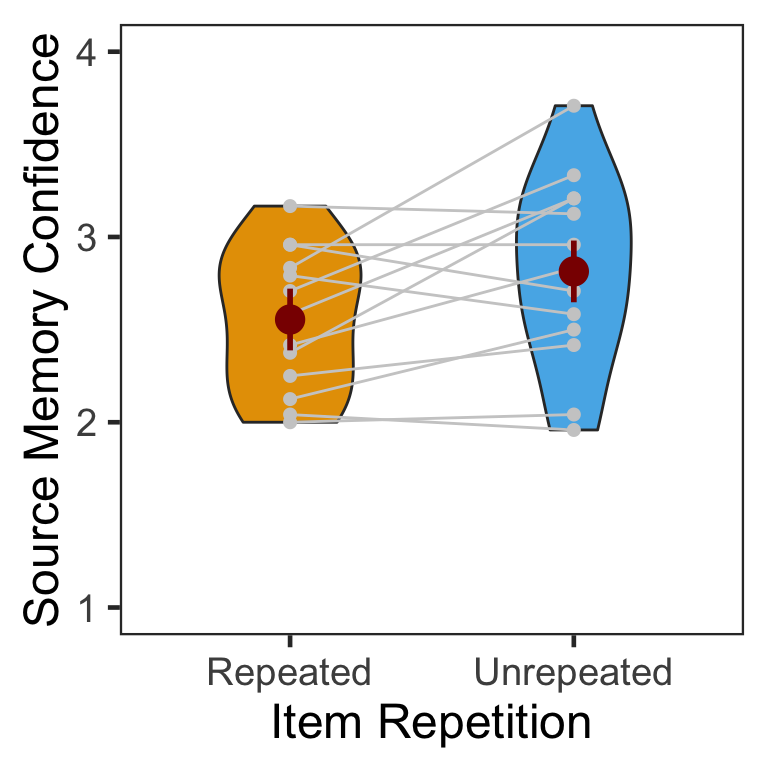### 4.2.1 ANOVA

Mean confidence ratings were submitted to a one-way repeated measures ANOVA.

ci95 <- P3CFswide %>%
mutate(Diff = Unrepeated - Repeated) %>%
summarise(lower = mean(Diff) - qt(0.975,df=n()-1)*sd(Diff)/sqrt(n()),
upper = mean(Diff) + qt(0.975,df=n()-1)*sd(Diff)/sqrt(n()))

p3.conf.aov <- aov_ez(id = "SID", dv = "Confidence", data = P3CFslong, within = "Repetition")
anova(p3.conf.aov, es = "pes") %>% kable(digits = 4)
num Df den Df MSE F pes Pr(>F)
Repetition 1 12 0.076 5.7662 0.3246 0.0334

Confidence ratings were higher for unrepeated than repeated faces. The 95% CI of difference between the means was [0.02, 0.5].

### 4.2.2 CLMM

The responses from a Likert-type scale are ordinal. Especially for the rating items with numerical response formats containing four or fewer categories, it is recommended to use categorical data analysis approaches, rather than treating the responses as continuous data (Harpe, 2015).

Here we employed the cumulative link mixed modeling using the clmm() of the package ordinal (Christensen, submitted). The specification of the full model was the same as the mixed() above.

P3R <- P3
P3R$Confident = factor(P3R$Confident, ordered = TRUE)
P3R$SID = factor(P3R$SID)

cm.full <- clmm(Confident ~ Repetition + (Repetition|SID) + (1|ImgName), data=P3R)
cm.red1 <- clmm(Confident ~ 1 + (Repetition|SID) + (1|ImgName), data=P3R) 

To determine the significance of the fixed effect, the LRT compared the full model (cm.full) with its nested model (cm.red1) without the effect of interest. The table below shows that item repetition significantly decreased confidence ratings.

cm.comp <- anova(cm.full, cm.red1)
data.frame(Effect = "Repetition", df = 1, Chisq = cm.comp$LR.stat, p = cm.comp$Pr(>Chisq)) %>% kable()
Effect df Chisq p
Repetition 1 7.069092 0.0078425

We plot the estimated marginal means, which were extracted from the fitted CLMM. It is evident that item repetition changed the distribution of confidence ratings.

temp <- emmeans(cm.full,~Repetition|cut,mode="linear.predictor")
temp <- rating.emmeans(temp)

colnames(temp) <- "Condition"

ggplot(data = temp, aes(x = Rating, y = Prob, group = Condition)) +
geom_line(aes(color = Condition), size = 1.2) +
geom_point(aes(shape = Condition, color = Condition),
size = 4, fill = "white", stroke = 1.2) +
scale_color_manual(values=c("#E69F00", "#E69F00")) +
scale_shape_manual(name="Condition", values=c(21,24)) +
labs(y = "Response Probability", x = "Rating",
group="Non-Famous Face") +
expand_limits(y=0) +
scale_y_continuous(limits = c(0, 0.5)) +
scale_x_discrete(labels = c("1" = "1(Guessed)","4"="4(Sure)")) +
theme_minimal() +
theme(text = element_text(size=18))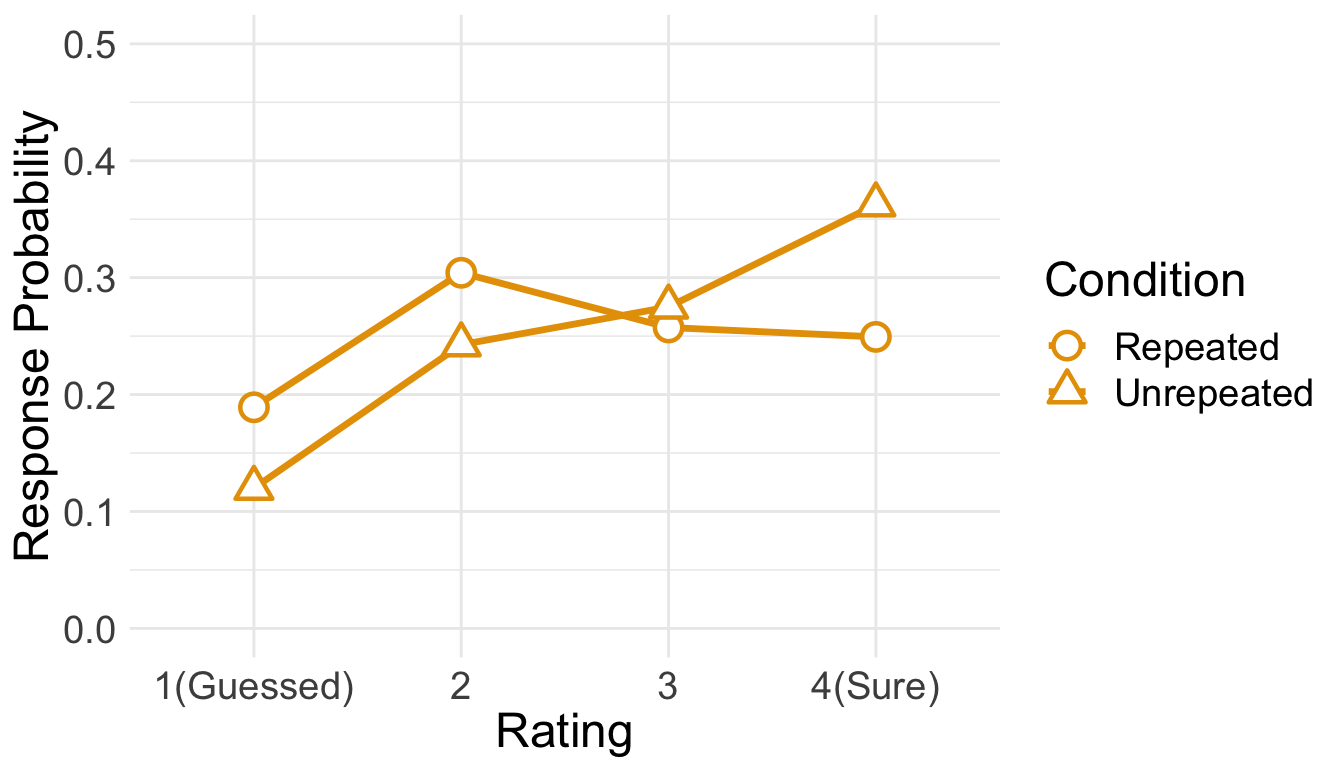## 4.3 RT

Only RTs from correct trials were analyzed. Before analysis, we first removed RTs either shorter than 200ms or longer than 10s. Then, from the RT distribution of each condition, RTs beyond 3 s.d. from the mean were additionally removed.

cP3 <- P3 %>% filter(Corr==1)

sP3 <- cP3 %>% filter(RT > 200 & RT < 10000) %>%
group_by(SID) %>%
nest() %>%
mutate(lbound = map(data, ~mean(.$RT)-3*sd(.$RT)),
ubound = map(data, ~mean(.$RT)+3*sd(.$RT))) %>%
unnest(lbound, ubound) %>%
unnest(data) %>%
ungroup() %>%
mutate(Outlier = (RT < lbound)|(RT > ubound)) %>%
filter(Outlier == FALSE) %>%
select(SID, Repetition, RT, ImgName)

100 - 100*nrow(sP3)/nrow(cP3)
##  2.419355

This trimming procedure removed 2.42% of correct trials.

Since the overall source memory accuracy was not high, only small numbers of correct trials were available after trimming. The following table summarizes the numbers of RTs submitted to subsequent analyses. No participant had more than 25 trials per condition.

sP3 %>% group_by(SID, Repetition) %>%
summarise(NumTrial = length(RT)) %>%
ungroup %>%
group_by(Repetition) %>%
summarise(Avg = mean(NumTrial),
Med = median(NumTrial),
Min = min(NumTrial),
Max = max(NumTrial)) %>%
ungroup %>%
kable()
Repetition Avg Med Min Max
Repeated 12.46154 11 9 19
Unrepeated 15.46154 16 5 23
den1 <- ggplot(cP3, aes(x=RT)) +
geom_density() +
theme_bw(base_size = 18) +
theme(panel.grid.major = element_blank(),
panel.grid.minor = element_blank(),
axis.text.y = element_blank(),
axis.ticks.y = element_blank())
den2 <- ggplot(sP3, aes(x=RT)) +
geom_density() +
theme_bw(base_size = 18) +
labs(x = "Trimmed RT") +
theme(panel.grid.major = element_blank(),
panel.grid.minor = element_blank(),
axis.text.y = element_blank(),
axis.ticks.y = element_blank())
den1 + den2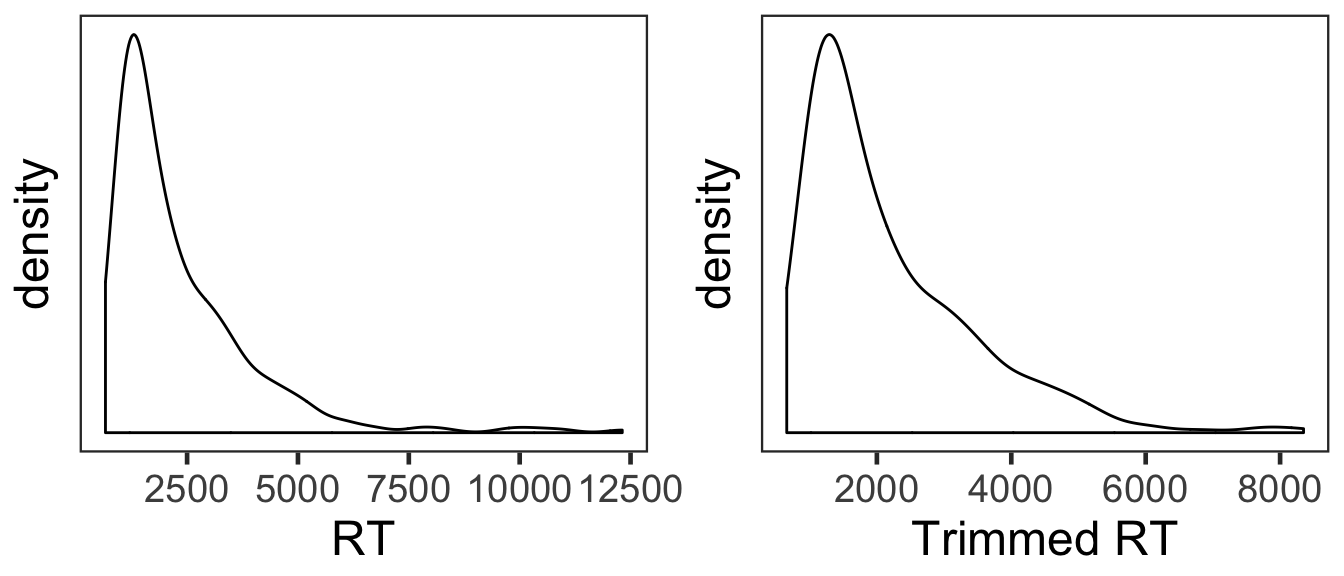The overall RT distribution was highly skewed even after trimming. Given limited numbers of RTs and its skewed distribution, any results from the current RT analyses should be interpreted with caution and preferably corroborated with other measures.

We calculated the mean and s.d. of individual participants’ mean RTs. The overall pattern of RTs was consistent with that of source memory accuracy and confidence ratings. Participants responded slightly faster in the unrepeated than repeated condition.

P3RTslong <- sP3 %>% group_by(SID, Repetition) %>%
summarise(RT = mean(RT)) %>%
ungroup()

P3RTg <- P3RTslong %>% group_by(Repetition) %>%
summarise(M = mean(RT), SD = sd(RT)) %>%
ungroup()
P3RTg %>% kable()
Repetition M SD
Repeated 2316.575 727.6523
Unrepeated 2224.606 904.5207
# wide format, needed for geom_segments.
P3RTswide <- P3RTslong %>% spread(key = Repetition, value = RT)

# group level, needed for printing & geom_pointrange
# Rmisc must be called indirectly due to incompatibility between plyr and dplyr.
P3RTg$ci <- Rmisc::summarySEwithin(data = P3RTslong, measurevar = "RT", idvar = "SID", withinvars = "Repetition")$ci
P3RTg$RT <- P3RTg$M

ggplot(data=P3RTslong, aes(x=Repetition, y=RT, fill=Repetition)) +
geom_violin(width = 0.5, trim=TRUE) +
geom_point(position=position_dodge(0.5), color="gray80", size=1.8, show.legend = FALSE) +
geom_segment(data=P3RTswide, inherit.aes = FALSE,
aes(x=1, y=P3RTswide$Repeated, xend=2, yend=P3RTswide$Unrepeated), color="gray80") +
geom_pointrange(data=P3RTg,
aes(x = Repetition, ymin = RT-ci, ymax = RT+ci, group = Repetition),
position = position_dodge(0.5), color = "darkred", size = 1, show.legend = FALSE) +
scale_fill_manual(values=c("#E69F00", "#56B4E9"),
labels=c("Repeated", "Unrepeated")) +
labs(x = "Item Repetition",
y = "Response Times (ms)") +
coord_cartesian(ylim = c(0, 5000), clip = "on") +
theme_bw(base_size = 18) +
theme(panel.grid.major = element_blank(),
panel.grid.minor = element_blank(),
legend.position = "none")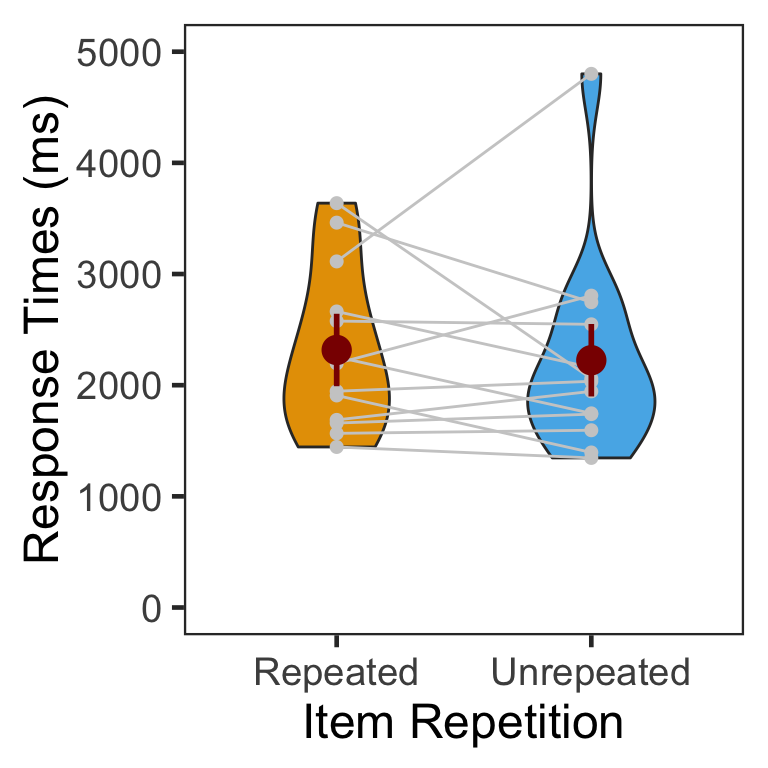### 4.3.1 ANOVA

Mean RTs were submitted to a one-way repeated measures ANOVA. The difference between conditions was not statistically significant.

p3.rt.aov <- aov_ez(id = "SID", dv = "RT", data = sP3, within = "Repetition")
anova(p3.rt.aov, es = "pes") %>% kable(digits = 4)
num Df den Df MSE F pes Pr(>F)
Repetition 1 12 290806.5 0.1891 0.0155 0.6714

# 5 Session Info

sessionInfo()
## R version 3.5.3 (2019-03-11)
## Platform: x86_64-apple-darwin15.6.0 (64-bit)
## Running under: macOS Mojave 10.14.4
##
## Matrix products: default
## BLAS: /Library/Frameworks/R.framework/Versions/3.5/Resources/lib/libRblas.0.dylib
## LAPACK: /Library/Frameworks/R.framework/Versions/3.5/Resources/lib/libRlapack.dylib
##
## locale:
##  en_US.UTF-8/en_US.UTF-8/en_US.UTF-8/C/en_US.UTF-8/en_US.UTF-8
##
## attached base packages:
##  parallel  stats     graphics  grDevices utils     datasets  methods
##  base
##
## other attached packages:
##   klippy_0.0.0.9500    patchwork_0.0.1      RVAideMemoire_0.9-73
##   ggbeeswarm_0.6.0     ordinal_2019.3-9     emmeans_1.3.3
##   afex_0.23-0          lme4_1.1-21          Matrix_1.2-17
##  car_3.0-2            carData_3.0-2        knitr_1.22
##  forcats_0.4.0        stringr_1.4.0        dplyr_0.8.0.1
##  tibble_2.1.1         ggplot2_3.1.0        tidyverse_1.2.1
##  Rmisc_1.5            plyr_1.8.4           lattice_0.20-38
##  pacman_0.5.1
##
## loaded via a namespace (and not attached):
##   TH.data_1.0-10      minqa_1.2.4         colorspace_1.4-1
##   rio_0.5.16          htmlTable_1.13.1    estimability_1.3
##   base64enc_0.1-3     rstudioapi_0.10     fansi_0.4.0
##  mvtnorm_1.0-10      lubridate_1.7.4     xml2_1.2.0
##  codetools_0.2-16    splines_3.5.3       Formula_1.2-3
##  jsonlite_1.6        nloptr_1.2.1        broom_0.5.1
##  cluster_2.0.7-1     compiler_3.5.3      httr_1.4.0
##  backports_1.1.3     assertthat_0.2.1    lazyeval_0.2.2
##  cli_1.1.0           acepack_1.4.1       htmltools_0.3.6
##  tools_3.5.3         lmerTest_3.1-0      coda_0.19-2
##  gtable_0.3.0        glue_1.3.1          reshape2_1.4.3
##  Rcpp_1.0.1          cellranger_1.1.0    nlme_3.1-137
##  xfun_0.5            openxlsx_4.1.0      rvest_0.3.2
##  MASS_7.3-51.3       zoo_1.8-5           scales_1.0.0
##  hms_0.4.2           sandwich_2.5-0      RColorBrewer_1.1-2
##  yaml_2.2.0          curl_3.3            gridExtra_2.3
##  rpart_4.1-13        latticeExtra_0.6-28 stringi_1.4.3
##  highr_0.8           ucminf_1.1-4        checkmate_1.9.1
##  boot_1.3-20         zip_2.0.1           rlang_0.3.3
##  pkgconfig_2.0.2     evaluate_0.13       htmlwidgets_1.3
##  labeling_0.3        tidyselect_0.2.5    magrittr_1.5
##  R6_2.4.0            generics_0.0.2      Hmisc_4.2-0
##  multcomp_1.4-10     pillar_1.3.1        haven_2.1.0
##  foreign_0.8-71      withr_2.1.2         survival_2.44-1
##  abind_1.4-5         nnet_7.3-12         modelr_0.1.4
##  crayon_1.3.4        utf8_1.1.4          rmarkdown_1.12
##  munsell_0.5.0       beeswarm_0.2.3      vipor_0.4.5